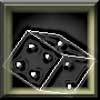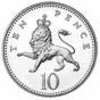#### You may also like### Gambling at Monte Carlo

A man went to Monte Carlo to try and make his fortune. Is his strategy a winning one?### Marbles and Bags

Two bags contain different numbers of red and blue marbles. A marble is removed from one of the bags. The marble is blue. What is the probability that it was removed from bag A?### Coin Tossing Games

You and I play a game involving successive throws of a fair coin. Suppose I pick HH and you pick TH. The coin is thrown repeatedly until we see either two heads in a row (I win) or a tail followed by a head (you win). What is the probability that you win?

##### Age 14 to 16Challenge Level

Thank you for this solution Andrei (Andrei Lazanu, School 205 Bucharest) and for the link to the useful site:

For a 2x2 grid I need to make 2 moves.

There are 4 possible paths:

left, left
left, up
up, left
up, up

Only 2 of these take me to the top left-hand corner of the grid, so the probability of getting to the opposite corner is: $${{1 \over{2^2}}\times2} = {2 \over4} = {1 \over2}$$

For a 3x3 grid I need to make 4 moves.

There are 16 possible paths.

Only 6 of these take me to the top left-hand corner of the grid, so the probability of getting to the opposite corner is: $${{1 \over{2^4}}\times6} = {6 \over16} = {3 \over8}$$

For a 4x4 grid I need to make 6 moves.

There are 64 possible paths.

Only 20 of these take me to the top left-hand corner of the grid, so the probability of getting to the opposite corner is: $${{1 \over{2^6}}\times20} = {20 \over64} = {5 \over16}$$

I found on the Internet, at the Math Forum, the formula together with the explanation.
The formula generating the number of ways to go from one corner to another is: $${[2(n-1)]!} \over{[(n-1)!]^2}$$
The formula generating the probability of landing in the opposite corner in a n x n grid is: $${{1 \over{2^{2(n-1)}}}} \times{{[2(n-1)]!} \over{[(n-1)!]^2}}.$$